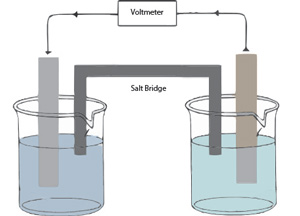# Electrochemistry

-Introduction

Step 1:

-Investigating redox reactions

-Practice with redox reactions

-Reduction tendencies of metal ions

Step 2:

-Electron transfer

-Electrochemical cell

-Practice with cells

-Powering a stopwatch

Step 3:

-Measuring potentials

-Calculating potentials

-Practice with potentials

-Applying potentials

Step 4:

-Non-standard conditions

-Practice with non-standard cells

Feedback Form:

Electrochemistry Tutorial: Galvanic Cells and the Nernst Equation >> Introduction

 Next >

# Electrochemistry: Galvanic Cells and the Nernst Equation

## IntroductionIn this experiment the goal is to investigate how to generate an electric current from a spontaneous redox chemical reaction. The device in which chemical energy is changed to electrical energy is called a galvanic cell. Batteries are an everyday example of galvanic cells.

In this experiment:

• We will build and investigate several galvanic cells.
• We will investigate the chemical reactions taking place in various galvanic cells and measure the cell potentials.
• We will study the electrodes that can be combined in various ways to form many different galvanic cells. We will determine the potential of these cells under standard conditions.
• We will investigate how the potential of the galvanic cell changes when we depart from the conditions defined as a "standard" and use the Nernst Equation to predict these changes.

The experimental strategy is shown below in the following 4 steps:

Step 1Action: Dip a strip of metal in a salt solution of another metal. Repeat with different metal/salt combinations.
Result: The observations provide evidence of whether or not a spontaneous reaction takes place. If a reaction is observed, we can use our knowledge of the chemical species involved to figure out the chemical reaction.

Step 2

Action: Create several half-cells, each consisting of a metal strip (an electrode) dipped in solution of that metal’s salt (an electrode solution). Combine various half-cells into pairs (galvanic cells) and try them out to power a stopwatch.
Result: We identify the galvanic cells which provide enough voltage to power a stopwatch.

Step 3

Action: Combine various half-cells into pairs (galvanic cells) and measure the voltage of the various pairs of half-cells.
Result: Create a series of electrode potentials arranged in order of the tendency of a metal ion to undergo reduction.

Step 4

Action: We measure voltage of a cell under standard conditions (1.0 M concentration). We change the concentration of one of the half-cells and observe the effect on the cell voltage. We repeat with various half-cell combinations.
Result: We determine the values of cell voltage under non-standard conditions.

The following pages contain tutors to assist you with understanding and applying some concepts required in the above steps. Please click next to continue to the online activity.

 Next >